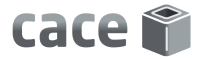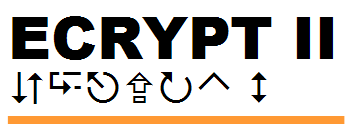# NaCl: Networking and Cryptography libraryComputer Aided Cryptography EngineeringECRYPT II
 Public-key cryptography: Authenticated encryption Scalar multiplication Signatures
 Secret-key cryptography: Authenticated encryption Encryption Authentication One-time authentication
 Low-level functions: Hashing String comparison

# Scalar multiplication: crypto_scalarmult

## C++ interface

C++ NaCl provides a crypto_scalarmult function callable as follows:
```     #include "crypto_scalarmult.h"

std::string p;
std::string n;
std::string q;

q = crypto_scalarmult(n,p);
```

This function multiplies a group element p by an integer n. It returns the resulting group element q of length crypto_scalarmult_BYTES. The function raises an exception if p.size() is not crypto_scalarmult_BYTES. It also raises an exception if n.size() is not crypto_scalarmult_SCALARBYTES.

C++ NaCl also provides a crypto_scalarmult_base function callable as follows:

```     #include "crypto_scalarmult.h"

std::string n;
std::string q;

q = crypto_scalarmult_base(n);
```

The crypto_scalarmult_base function computes the scalar product of a standard group element and an integer n. It returns the resulting group element q of length crypto_scalarmult_BYTES. It raises an exception if n.size() is not crypto_scalarmult_SCALARBYTES.

## C interface

C NaCl provides a crypto_scalarmult function callable as follows:
```     #include "crypto_scalarmult.h"

const unsigned char p[crypto_scalarmult_BYTES];
const unsigned char n[crypto_scalarmult_SCALARBYTES];
unsigned char q[crypto_scalarmult_BYTES];

crypto_scalarmult(q,n,p);
```

This function multiplies a group element p, ..., p[crypto_scalarmult_BYTES-1] by an integer n, ..., n[crypto_scalarmult_SCALARBYTES-1]. It puts the resulting group element into q, ..., q[crypto_scalarmult_BYTES-1] and returns 0.

C NaCl also provides a crypto_scalarmult_base function callable as follows:

```     #include "crypto_scalarmult.h"

const unsigned char n[crypto_scalarmult_SCALARBYTES];
unsigned char q[crypto_scalarmult_BYTES];

crypto_scalarmult_base(q,n);
```

The crypto_scalarmult_base function computes the scalar product of a standard group element and an integer n, ..., n[crypto_scalarmult_SCALARBYTES-1]. It puts the resulting group element into q, ..., q[crypto_scalarmult_BYTES-1] and returns 0.

## Representation of group elements

The correspondence between strings and group elements depends on the primitive implemented by crypto_scalarmult. The correspondence is not necessarily injective in either direction, but it is compatible with scalar multiplication in the group. The correspondence does not necessarily include all group elements, but it does include all strings; i.e., every string represents at least one group element.

## Representation of integers

The correspondence between strings and integers also depends on the primitive implemented by crypto_scalarmult. Every string represents at least one integer.

## Security model

crypto_scalarmult is designed to be strong as a component of various well-known "hashed Diffie–Hellman" applications. In particular, it is designed to make the "computational Diffie–Hellman" problem (CDH) difficult with respect to the standard base.

crypto_scalarmult is also designed to make CDH difficult with respect to other nontrivial bases. In particular, if a represented group element has small order, then it is annihilated by all represented scalars. This feature allows protocols to avoid validating membership in the subgroup generated by the standard base.

NaCl does not make any promises regarding the "decisional Diffie–Hellman" problem (DDH), the "static Diffie–Hellman" problem (SDH), etc. Users are responsible for hashing group elements.

## Selected primitive

crypto_scalarmult is the function crypto_scalarmult_curve25519 specified in "Cryptography in NaCl", Sections 2, 3, and 4. This function is conjectured to be strong. For background see Bernstein, "Curve25519: new Diffie-Hellman speed records," Lecture Notes in Computer Science 3958 (2006), 207–228, https://cr.yp.to/papers.html#curve25519.

## Alternate primitives

NaCl supports the following scalar-multiplication functions:
crypto_scalarmultBYTESSCALARBYTES
[TO DO:] crypto_scalarmult_nistp2566432
crypto_scalarmult_curve255193232
For example, a user who wants to use the Curve25519 group can replace crypto_scalarmult, crypto_scalarmult_BYTES, etc. with crypto_scalarmult_curve25519, crypto_scalarmult_curve25519_BYTES, etc.

## Version

This is version 2016.03.15 of the scalarmult.html web page.